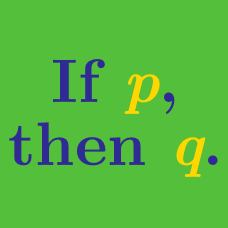Everyday Math

# Trial and Error

At Memorial Park, there are 3 times as many children as there are dogs. If $A$ is the number of children and $B$ is the number of dogs, which of the following are possible values for $A$ and $B?$

(A) $\ \ A =30, B = 3$
(B) $\ \ A=30, B = 10$
(C) $\ \ A =10, B = 30$
(D) $\ \ A =10, B = 3$
(E) $\ \ A =3, B = 10$

If $6x - 18 = 0,$ which of the following is the value of $x?$

(A) $\ \ 2$
(B) $\ \ 3$
(C) $\ \ 4$
(D) $\ \ 5$
(E) $\ \ 6$

If the sum of two numbers is $15$ and the difference of the two numbers is $9,$ what are the two numbers?

(A) $\ \ 11$ and $4$
(B) $\ \ 11$ and $5$
(C) $\ \ 12$ and $3$
(D) $\ \ 13$ and $2$
(E) $\ \ 13$ and $4$

Which of the following is the smallest?

(A) $\ \ \frac{1}{5} \div \frac{1}{8}$

(B) $\large \frac{1}{8} \div \frac{1}{5} \div 8$

(C) $\large \frac{1}{\frac{1}{5 \div 8}}$

(D) $\ \ 8 \div \frac{1}{5}$

(E) $\large \ \frac{1}{\frac{1}{5} \div \frac{1}{8}}$

If the sum of the consecutive odd integers from $x$ to $3$ (both inclusive) is $-21,$ which of the following is the value of $x$?

(A) $\ \ -3$
(B) $\ \ -5$
(C) $\ \ -7$
(D) $\ \ -9$
(E) $\ \ -11$

×